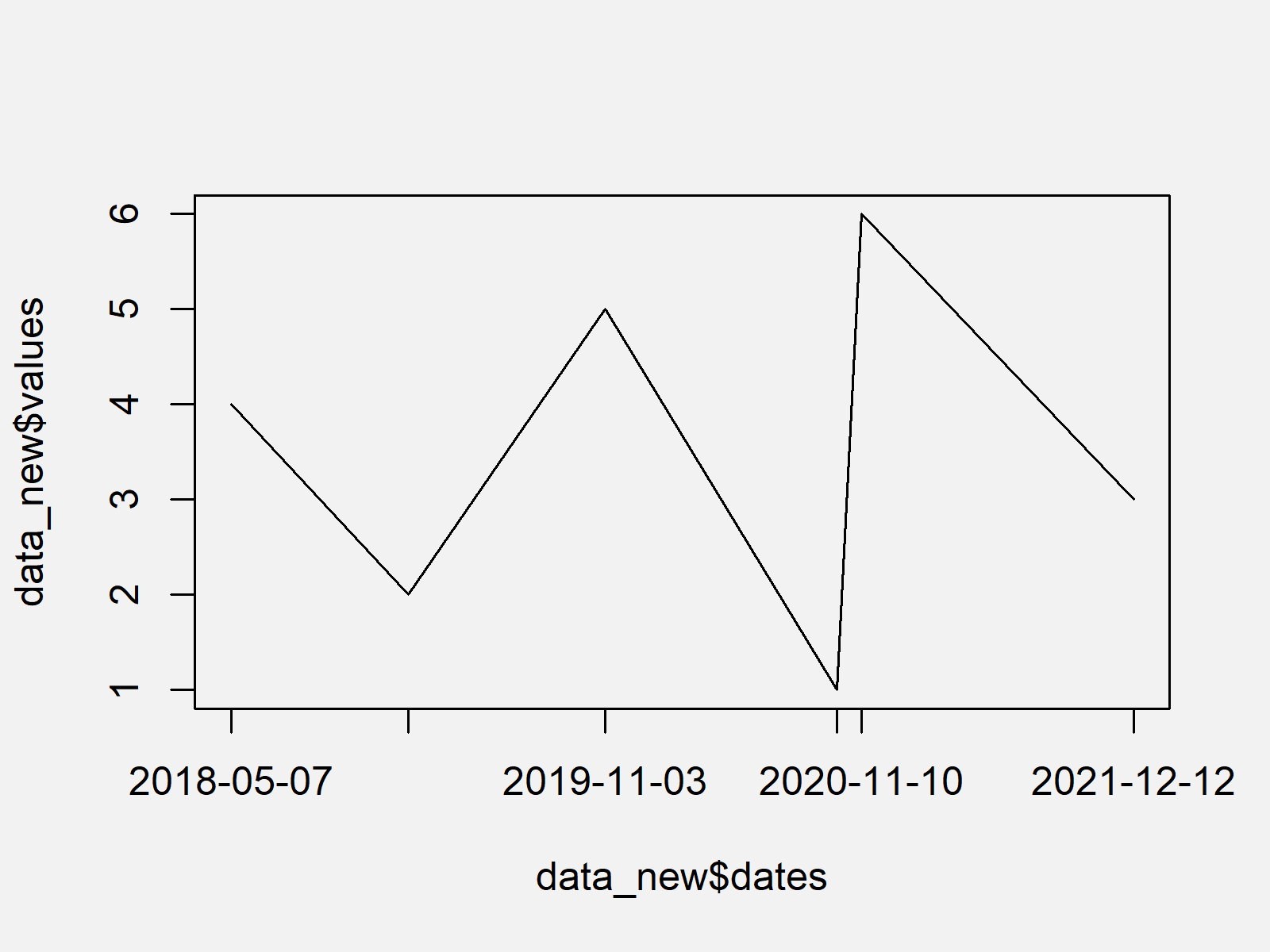# Draw Dates to X-Axis of Plot in R (2 Examples) | Time Series in Base R & ggplot2 Graph

In this R tutorial you’ll learn how to draw dates on a time series plot.

So now the part you have been waiting for – the examples.

## Creating Example Data

First, we need to create some data that we can use in the exemplifying code below:

```data <- data.frame(dates = c("2020-10-05", "2019-01-20",  # Create example data
"2021-12-12", "2018-05-07",
"2019-11-03", "2020-11-10"),
values = 1:6)
data                                                      # Print example data
#        dates values
# 1 2020-10-05      1
# 2 2019-01-20      2
# 3 2021-12-12      3
# 4 2018-05-07      4
# 5 2019-11-03      5
# 6 2020-11-10      6```

The previous output of the RStudio console shows that our example data has six rows and two columns. The first column contains our dates and the second variable consists of numeric values.

## Example 1: Plotting Dates on X-Axis in Base R Plot

Example 1 illustrates how to draw a plot with dates on the x-axis using the basic installation of the R programming language.

For this, we first have to modify our data so that the dates column has the class Date. Furthermore, we have to order our new data frame according to the dates:

```data_new <- data                                          # Duplicate data
data_new\$dates <- as.Date(data_new\$dates)                 # Convert to Date object
data_new <- data_new[order(data_new\$dates), ]             # Order data
data_new                                                  # Print updated data
#        dates values
# 4 2018-05-07      4
# 2 2019-01-20      2
# 5 2019-11-03      5
# 1 2020-10-05      1
# 6 2020-11-10      6
# 3 2021-12-12      3```

Now, we can use the plot and axis functions to draw a graph with dates (i.e. year, month, and day) on the x-axis as shown below:

```plot(data_new\$dates,                                      # Draw plot without x-axis
data_new\$values,
type = "l",
xaxt = "n")
axis(1,                                                   # Add dates to x-axis
data_new\$dates,
format(data_new\$dates, "%Y-%m-%d"))```As shown in Figure 1, we created a line chart with dates on the x-axis with the previously shown syntax.

## Example 2: Plotting Dates on X-Axis of ggplot2 Plot

The following R code shows how to create a ggplot2 plot with dates on the axis of our time series.

We first have to install and load the ggplot2 package to R, if we want to use the functions and commands that are included in the package:

```install.packages("ggplot2")                               # Install ggplot2 package

Now, we can use the following R code to print our plot:

```ggplot(data_new, aes(x = dates, y = values)) +            # Draw ggplot2 plot
geom_line() +
scale_x_date(date_labels = "%Y-%m")```Figure 2 shows the output of the previous syntax: A ggplot2 graphic with dates on the x-axis. Note that we can use the scale_x_date function to modify the way our dates are shown.

## Video, Further Resources & Summary

I have recently published a video on my YouTube channel, which shows the R programming codes of this article. You can find the video below:

Please accept YouTube cookies to play this video. By accepting you will be accessing content from YouTube, a service provided by an external third party.If you accept this notice, your choice will be saved and the page will refresh.

Also, you could have a look at the other tutorials on my website:

Summary: At this point you should know how to plot dates on an x-axis in R. Don’t hesitate to let me know in the comments section, if you have any additional questions.

Subscribe to the Statistics Globe Newsletter